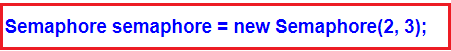# Semaphore in C#

## Semaphore in C# with Example

In this article, I am going to discuss how to use Semaphore in C# with example. Please read our previous article where we discussed how to use Monitor in C# to protect the shared resources from concurrent access in a multithread application. As part of this article, we are going to discuss the following pointers which are related to C# semaphore.

1. What is Semaphore in C#?
2. How does Semaphore work in C#?
3. How to use the Semaphore class?
4. Understanding the different methods of Semaphore class with examples.
##### What is Semaphore in C#?

The Semaphore in C# is used to limit the number of threads that can have access to a shared resource concurrently. In other words, we can say that Semaphore allows one or more threads to enter into the critical section and execute the task concurrently with thread safety. So, in real-time, we need to use Semaphore when we have a limited number of resources and we want to limit the number of threads that can use it.

##### How does Semaphore work in C#?

The Semaphores are Int32 variables that are stored in operating system resources. When we initialize the semaphore object we initialize it with a number. This number basically used to limits the threads that can enter into the critical section.

So, when a thread enters into the critical section, it decreases the value of the Int32 variable with 1 and when a thread exits from the critical section, it then increases the value of the Int32 variable with 1. The most important point that you need to remember is when the value of the Int32 variable is 0, then no thread can enter into the critical section.

##### The syntax for semaphore initialization in C#:

You can use the following statement to create the Semaphore instance in C#.As you can see in the above statement, we are passing two values to the constructor of the Semaphore class while initializing. These two values represent InitialCount and MaximumCount.

The InitialCount parameter sets the value for the Int32 variable. that is it defines the initial number of requests for the semaphore that can be granted concurrently. MaximumCount parameter defines the maximum number of requests for the semaphore that can be granted concurrently.

For example, if we set the maximum count value as 3 and initial count value 0, it means 3 threads are already in the critical section. If we set the maximum count value as 3 and the initial count value 2. It means a maximum of 3 threads can enter into the critical section and there is one thread that is currently in the critical section.

Note: The second parameter always must be equal or greater than the first parameter otherwise we will get an exception.

##### Methods of Semaphore class:

WaitOne Method: Threads can enter into the critical section by using the WaitOne method. We need to call the WaitOne method on the semaphore object. If the Int32 variable which is maintained by semaphore is greater than 0 then it allows the thread to enter into the critical section.

Release Method: We need to call the Release method when the thread wants to exits from the critical section. When this method is called, it increments the Int32 variable which is maintained by the semaphore object.

##### Let us see an example for a better understanding.
```using System;

namespace MutexDemo
{
class Program
{
public static Semaphore semaphore = new Semaphore(2, 3);

static void Main(string[] args)
{
for (int i = 1; i <= 10; i++)
{
{
Name = "Thread " + i
};
}
}

{

try
{
semaphore.WaitOne();